Three Phase Systems

4964 words (20 pages) Essay in Engineering

31/08/17 Engineering Reference this

Disclaimer: This work has been submitted by a student. This is not an example of the work produced by our Essay Writing Service. You can view samples of our professional work here.

Any opinions, findings, conclusions or recommendations expressed in this material are those of the authors and do not necessarily reflect the views of UK Essays.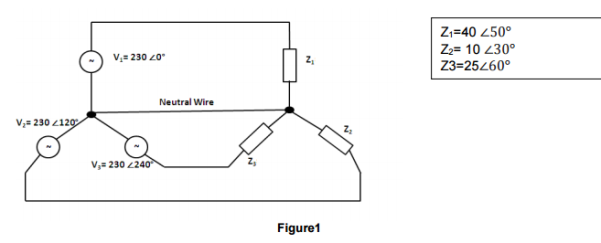Determine the neutral current in the unblanced 4-wire star power system shown in figure1. Express the answer in both complex number and polar forms.

Use a simulator to confirm the magnitude of the nuetral current which is eqaluated.

Given that,

Z1 = 40 âˆ 50Â°

Z2 = 10 âˆ 30Â°

Z3 = 25 âˆ 60Â°

V1 = 230 âˆ 0Â°

V2 = 230 âˆ 120Â°

V3 = 230 âˆ 240Â°

ï‚§To Find Neutral Current by Calculation:

To find the neutral current, first we have to find I1, I2 and I3 and then add them together to get the neutral current.

ï‚§To find I1:

I1 =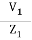I1 =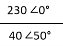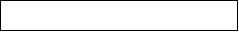I1 =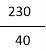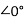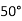I1 = (5.75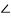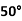) A (Polar Form)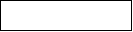Current in Rectangular Form:

I1 = (3.69 -j 4.40) A

ï‚§To find I2:

I2 =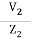I2 =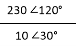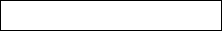I2 =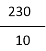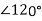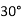I2 = (23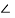90) A (Polar Form)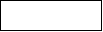Current in Rectangular Form:

I2 = (0 +j 23) A

ï‚§To find I3:

I3 =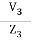I3 =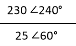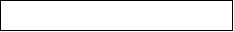I3 =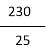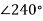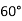I3 = (9.2180) A (Polar Form)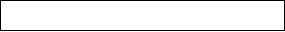I3 = (-9.2 +j 0) A (Rectangular Form)

Now to find neutral current IN, we need to add I1, I2 and I3

IN = I1 + I2 + I3

IN = (3.69 -j 4.40) + (0 +j 23) + (-9.2 +j 0)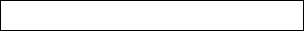IN = (3.96 + 0 – 9.2) +j (-4.40 + 23 + 0)

IN = (-5.51 +j 18.6) A (Rectangular Form)IN = 19.39106.50A (Polar Form)

ï‚§Using TINA Simulation: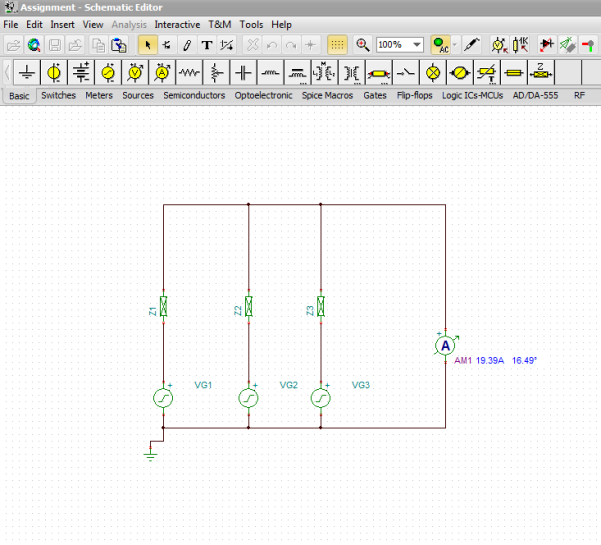Use the three-wattmeter method on a simulator to determine the total effective power dissipated in Figure1. Confirm this answer by calculation.

ï‚§Using TINA Simulation: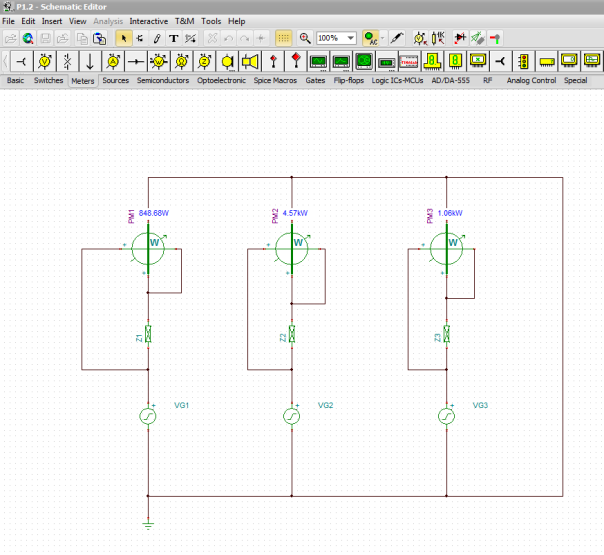ï‚§Total Effective Power Dissipated in Figure1 by Calculation:

To find total effective power dissipated, first we have to find P1 eff, P2 effand P3 eff

ï‚§To find P1 eff :

P1 eff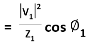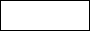P1 eff =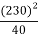cos 50P1 eff = 850 W

ï‚§To find P2 eff :

P1 eff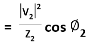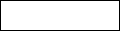P2 eff =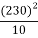cos 30P2 eff = 4581.27 W

ï‚§To find P3 eff :

P1 eff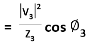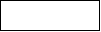P1 eff =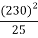cos 60P1 eff = 1058 W

P total = P1 eff + P2 eff+ P3 eff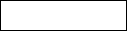P total = 850 + 4581.27 + 1058

P total = 6489.27 W

If V1 went open circuit, calculate the new value of neutral current for Figure1.

if V1 went open or is at fault then I1 will become zero and the neutral current will be the sum of the other two currents I1 and I2

IN = I1 + I2 + I3

V1 went open so, I1 = 0 therefore,

IN = 0 + I2 + I3

IN = I2 + I3

IN = (0 +j 23) + (-9.2 +j 0)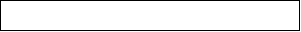IN = (0 – 9.2) + j (23 + 0)

IN = (-9.2 + j23) A (Rectangular Form)IN = 24.77111.80A (Polar Form)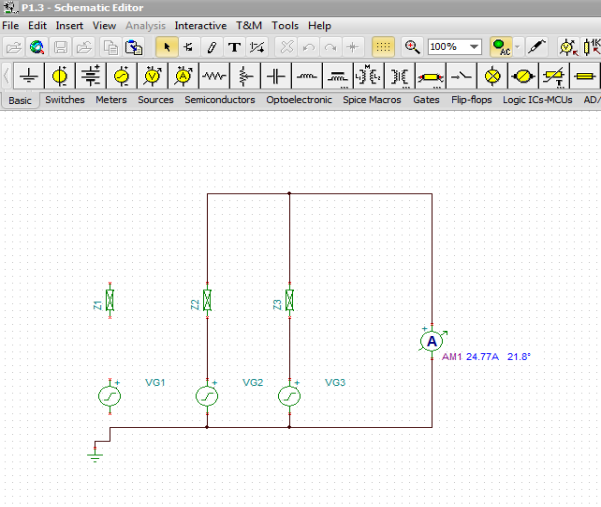Single-core Three-Phase Double-Wound Transformer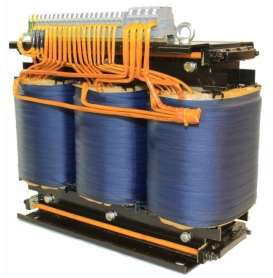Introduction:

Transformer is a static device which transfers electrical energy or power between two or more than two circuits using phenomena of Electromagnetic induction. It takes the current of one voltage and converts it into a different voltage. The amount of transferred energy from Primary circuit to the Secondary Circuit is equal, and the losses are ignored. The frequency of the circuits does not change but the voltage and current of each of the individual circuit is changed, it is increased or decreased, unless we use the transformer as a one-to-one isolating transformer.

Basically, the transformer perform the function of changing electricity from high to low voltage by making use of the following properties of electricity.

• Magnetism is always present around in an electric circuit.
• Magnetic field is always changed by changing the strength or moving the conductor therefore voltage is induced.

Voltage is known as the measure of the “Force” or “Pressure” that is used to push the electrons around the circuit.

Electricity at a higher voltage goes in the transformer and runs through the large number of coils that are wounded around the iron core. As the current is changing, the core magnetism will also be changing. A wire with less coils around the iron core is known as the output wire and the changing magnetism produces current in the wire. When the number of coils are less that means less voltage and more coils means more voltage. Therefore, the voltage is either stepped down or stepped up according to the number of coils in the wire.

A common transformer involves a ferromagnetic core which ensures that values of magnetic flux linkage remain high. But there are some factors related to the ferromagnetic materials that are responsible for part of the loss associated with power transfer. These losses are as follows:

1.Core losses:

Core losses can be classified as the sum of eddy currents losses and the hysteresis loss.

• Hysteresis loss:

Energy that a magnetic material soaks in cannot be recovered completely. Core of the material is excited by an A.C source, the flux density rises and falls with the magnetic field strength or current which is basically sinusoidal. When the magnetizing current increases the flux density also increases but there comes a point after which if there is any further increase in the current, flux density is saturated. If the current is reduced from the saturation point to zero, flux density will start to decrease. When the value of the current reaches upto zero the flux density should also become zero but it does not become equal to zero. Some of the flux density will still be present in the material for the zero current, which is called as a residual magnetic flux. Thus, the total amount of energy used to magnetize the material cannot be recovered back and this energy is trapped within the material core and is dissipated as a form of heat. Area of B/H curve gives the hysteresis loss, larger the loop the greater the energy required to create the magnetic field.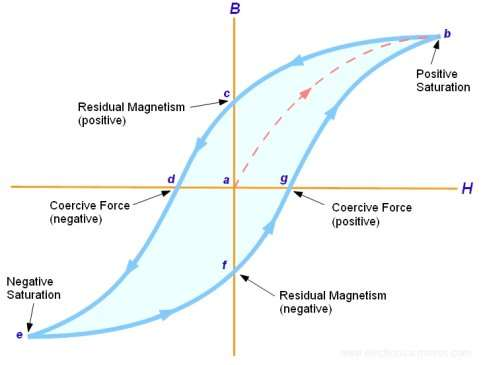• Eddy current losses:

If a coil is wrapped around a core made up of some material and an A.C source is applied to circuit, eddy current losses take place. As the supply to the coil is changing or alternating which produces an alternating flux in the coil. The varying flux in the core induces e.m.f in the core material due to which eddy currents start to flowing in the core material. Therefore, the energy is lost or dissipated in the form of heat because of eddy currents.

The laminations in the core can reduce the eddy current losses. Thin sheet steels which are separated from each other must be used because these insulated sheets reduce the amount of the current flow and hence the eddy current losses.

2.Copper losses:

Copper loss occurs when the transformer is loaded, the input power is dissipated as heat in the primary and secondary windings of the transformer because these windings have internal resistance. When the load is low, the amount of heat produced is small but at high loads the amount of heat produced becomes significant.

ï‚·Construction of Single-core three-phase double wound Transformer: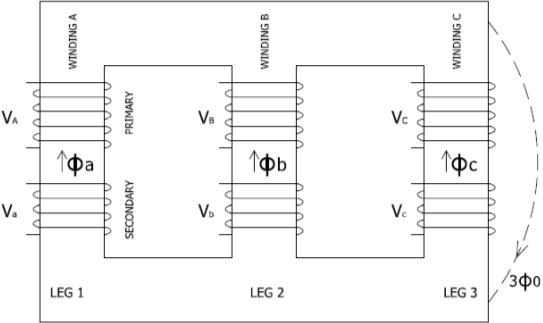A single-core three-phase double wound transformer consists of three sets of primary and secondary windings, individual set winded on each leg of the core made up of iron. The windings consists of turns made up with the insulated wire. These coils are then mounted on a magnetic core material in such a way that the magnetic flux produced by one coil increases and ensures that the most of the magnetic flux links with the second coil so that mutual induction is being increased. The coils are spaced 120 degrees apart. The transformer is filled with a dielectric oil and thus provide insulation in-between the winding and case. These windings are either connected in star or delta configurations.

• Delta Connection:

Delta system proves excellent for distribution system for short distances. These types of transformers are used in neighbourhood and commercial loads of small magnitude near to the substations. There is one kind of voltage present between the 2 wires in the delta system. This system is shown by a Triangle. The voltage between any two wires will be the same and from each individual point of the Triangle, wire emerges which represents a three phase.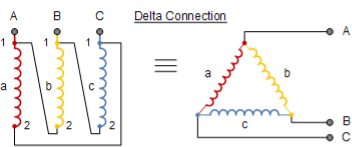• Star Connections:

For a star connection, voltage of two lines is the same as the voltage on a 3 phase system. In Star connection, each of the windings are made up connecting each individual winding end to end and this point is the “star point” or “neutral point” of connection winding. Connection for neutral and 3 phase connections are then taken out of transformer.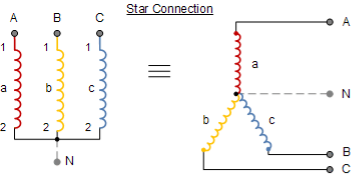ï‚·Applications of Transformers:

• Transformers in Electrical Engineering:

An electrical engineer may put a transformer in a circuit for many reasons. If the device being powered require higher power than what is available locally or the powered device needs frequency matching, a transformer is placed in such circuits. For example signal and audio transformers are used to couple amplifiers and microphones to the input of the amplifiers.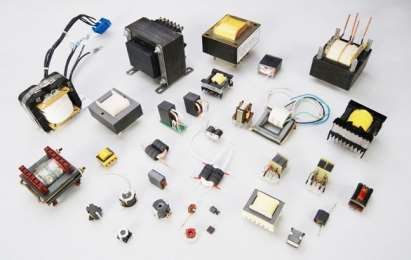• Power Utilities:

When driving through a rural area, power polls and overhead power lines can be seen and transformers are placed on these power polls by power utilities. Utility substations make use of transformers to deliver power of certain usable level and quality to consumers and businesses.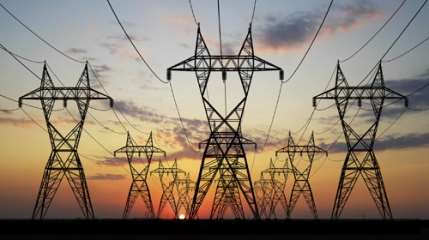• Power Generation and Distribution:

Electrical power is generated at the power station and this electrical energy is then transferred to the consumers through high tension power lines. To distribute the electricity from the power station to the consumer, different transformers are used in stepping up and stepping down the voltage up to the certain level.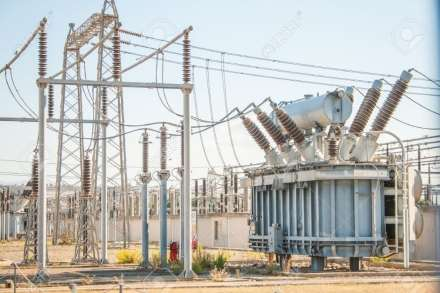• Commercial use:

Every electrical device uses transformer in one way or another. Almost every commercial building has at least three or four large high power transformers in order to provide required voltages to operate overhead lightning system, telephone system, heating, operating electrical equipment in labs and much more.

• Transformers in home: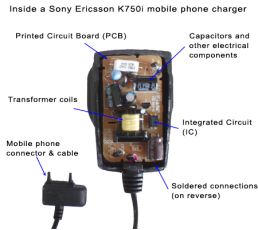There are a lot of transformers used in many appliances at our homes that require different voltages for their operation. Electric appliances like dishwasher, washing machine, microwave and fridge require comparatively high voltages between 110 and 240 volts, the devices like laptops and charger mobile phones and MP3 players require relatively low voltages. A laptop charger requires up to 15V while mobile phone charger requires up to 6V and M3 chargers require up to 12V to charge up their batteries. Thus, these appliances have small transformers built in them which convert the domestic supply of 110-240 volts into a low voltage that they require for their operation.

ï‚·Power Transmission and Distribution System: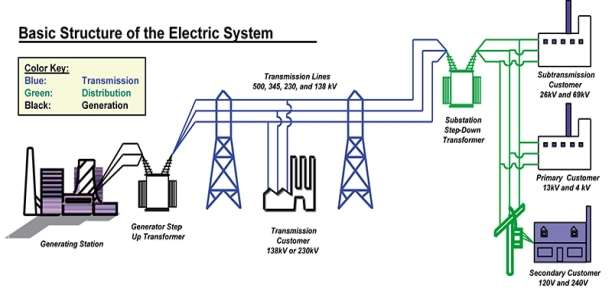Three-phase system is used in power transmission because less amount of conductor is used to transmit electrical energy to different places.

Three conductors carry 3 alternating currents peak values of which reach at different intervals of time. If a conductor is taken as a reference point, there is a delay of time by one third and two third of a cycle of the current for the remaining two currents. Because of this delay in the available phases, constant power is transferred over each cycle of current.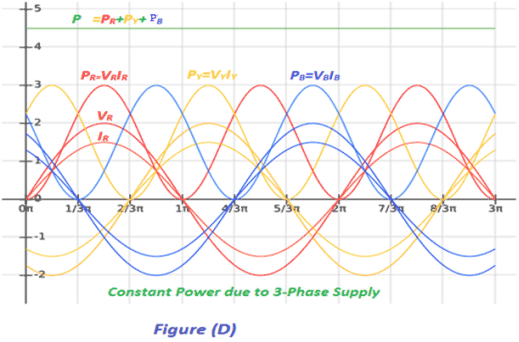There can or cannot be a neutral wire in three-phase systems. Neutral wire is used to allow a higher voltage while still supporting the appliances operating at lower single phase voltages. As loads can directly be connected between the phases so there is no neutral current wire in high voltage distribution.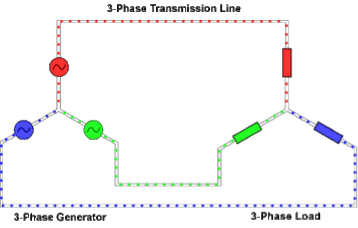There are many factors that make three-phase system very desirable and reliable in electric power systems. In a load which is linearly balanced, the phase currents cancel each other and add up to give a zero which also helps in eliminating the neutral wire. Phase conductor carry same amount of current which means that they all can have a same size of conductor for balanced conditions. Moreover, the transfer of power in the system is constant for a linear system, it reduces the vibrations in a system.

ï‚·Generation:

Electricity is generated at the power station at around 10kV by rotating a coil in the magnetic field. In power stations, all the generators are synchronised so that they all produce electricity that oscillates together. For more efficient generation, three windings are used on the generator to produce three-phase electricity.

Sources for power generation:

For distribution, the following types of resources are available for the generation of the electricity.

• Conventional Methods: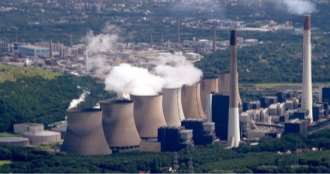1. Thermal energy:

Thermal energy or the Nuclear energy is used to produce steam for the turbines that drives the rotating AC generators to produce electrical energy.

1. Hydro-electric:

Potential of water is stored at a higher altitude and is made passed through the turbines which in turn drive the generators.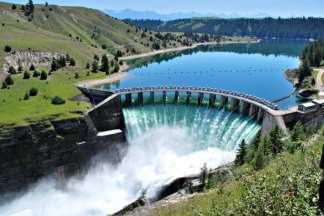• Non-Conventional Methods:

Wind power:

Winds blowing at high speeds are used to drive the turbines that are coupled to the rotating AC generators.ï‚·Transmission:

Transmission has an important role between the production of power and the usage of power. Transmission lines carry high voltage electricity over long distances from power plants to the consumers. Once the power is generated. It is send to the substation in the power plant where the voltage is stepped-up using step up transformer for transmission purposes.

When the voltage is stepped up, it helps in the reduction of the transmission losses. It is then sent to the grid station from where it is further transmitted to different cities.

• Why there is a need to step up the voltage for transmission:

For transmission over long distances, transmission lines are made up of conducting materials like aluminium and power loss is always associated with these conducting materials. This is because if the current through the wire is “I” and the resistance of the wire is “R” then an amount of electrical power “I2R” is dissipated as heat. This will result in a much less power at the receiving end than the actual power generated at the station.

Thus, when power is transmitted at a high voltage, wires of low current carrying capacity are used so there is no need of thick wires that cost considerably high instead they can be replaced by thin wires that cost less.

ï‚·Distribution:

Substations reduce the voltage of the electricity from the transmission lines. The power grid is connected to the different parts of the country and it distributes the power in different cities through transmission lines.

The transmitted power from the power grid is stepped-down to 110,000 volts from 230,000 volts using a step-down auto transformer. Then for the distribution of power for industrial uses, the power is again stepped-down to 11,000 volts from 110.000 volts by step-down transformer located in the streets. This reduction in the voltage increases the current in the wires and thus the losses but greatly reduces the cost of power poles and increases the safety.

The distribution substation further reduces the voltage to 230 volts or 440 volts for the local transmission for domestic purposes depending upon the need of the user. Some business and houses require two or three-phase power supply while the others only need single phase supply.

ï‚·Operation of the transformer:

Transformer works on the principle of mutual induction. According to Faraday’s Law of electromagnetic induction:

“Rate of change of flux linkage is directly proportion to the induced emf in a conductor or coil with respect to time.”

When primary winding is connected to a voltage source, an AC flows through it and which produces a continually changing magnetic flux that surrounds the winding. This changing magnetic flux flows to the secondary winding as some flux from primary winding will be linked to the secondary winding. As magnitude and direction of the flux is continually alternating, change of flux takes place in the secondary winding. According to Faraday’s law of electromagnetic induction, this changing magnetic flux produces an induced “electro motive force” or simply the “voltage” in the secondary winding of transformer therefore, a current start to flow if the circuit is closed.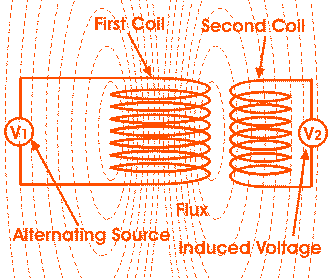The rate at which the flux changes depends upon the amount of the flux which is linked with the secondary winding of the transformer. Therefore, it is important to link all the flux from the primary winding to the secondary winding of the transformer. It can be done by putting a low reluctance path which is usually common to both of the windings and that low reluctance path is known as the core of the transformer.

Three-phase Induction Motor

Introduction:

Electric motor is a device which performs the operation of converting electrical energy into a mechanical energy. The induction motor is known as a three-phase AC motor which is widely used in the industrial applications as they are:

• Self-starting.
• Their construction is simple and compact.
• Less expensive.
• Require minimum amount of maintenance.
• More reliable and highly efficient.
• Additional starting motor is not required and do not required to be synchronised.

Induction motor is also known as asynchronous motor. Due to inductive coupling, the generated electromagnetic power is transferred to the secondary winding. These windings are being separated by air gap between them.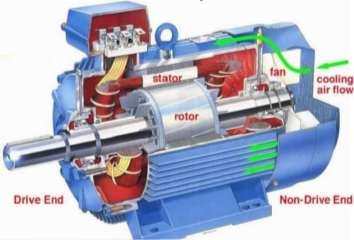The induction motor consists of a Stator and a Rotor. The motor frame is permanently attached with windings of very low resistance. When current and voltage is applied to the terminals of Stator windings, a magnetic field is produced.

ï‚·Applications of three-phase induction motor:

Induction motors are most demanded motors in the industry. Some of their applications are given below:

• Industrial drives make use of 3 phase cage rotor motors because they are pretty simple, efficient and are not costly.
• Single phase induction motors are used where the load is comparatively smaller.
• These motors are being

Related Services

View all

DMCA / Removal Request

If you are the original writer of this essay and no longer wish to have the essay published on the UK Essays website then please.Prices from
£28

Undergraduate 2:2 • 250 words • 7 day delivery

Delivered on-time or your money back

Rated 4.6 out of 5 by(119 Reviews)